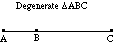index: click on a letter A B C D E F G H I J K L M N O P Q R S T U V W X Y Z A to Z index index: subject areas numbers & symbols sets, logic, proofs geometry algebra trigonometry advanced algebra & pre-calculus calculus advanced topics probability & statistics real world applications multimedia entrieswww.mathwords.com about mathwords website feedback

 Degenerate An example of a definition that stretches the definition to an absurd degree. A degenerate triangle is the "triangle" formed by three collinear points. It doesn’t look like a triangle, it looks like a line segment. A parabola may be thought of as a degenerate ellipse with one vertex at an infinitely distant point. Degenerate examples can be used to test the general applicability of formulas or concepts. Many of the formulas developed for triangles (such as area formulas) apply to degenerate triangles as well.See also Degenerate conics